# Single Phase Power

Single phase power refers to the distribution system in which; all the voltages vary in unison. It can be generated simply by rotating a moving coil in a magnetic field or by moving field around a stationary coil. The alternating voltage and alternating current so produced, thus referred to as single phase voltage and current.

Different types of circuits show different response to the application of sinusoidal input. We will consider all type of circuits one by one that include electrical resistance only, capacitance only and inductor only, and a combination of these three and try to establish single phase power equation.

### Equation for Purely Resistive Circuit

Let’s examine single phase power calculation for purely resistive circuit. Circuit consisting of pure ohmic resistance is across a voltage source of voltage V, is shown below in the figure.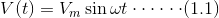Where, V(t) = instantaneous voltage.
Vm = maximum value of voltage.
ω = angular velocity in radians/seconds.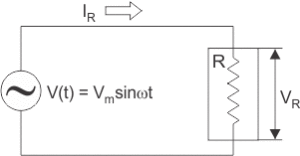According to Ohm’s law ,Substituting value of V(t) in above equation we get,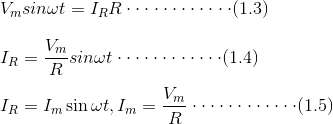From equations (1.1) and (1.5) it is clear that V(t) and IR are in phase. Thus in case of pure ohmic resistance, there is no phase difference between voltages and current, i.e. they are in phase as shown in figure (b).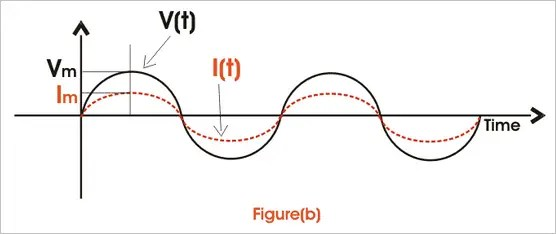Instantaneous power,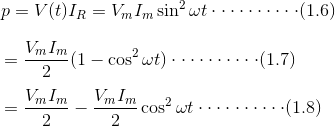From single phase power equation (1.8) it is clear that power consist of two terms, one constant part i.e.and another a fluctuating part i.e.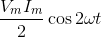That’s value is zero for the full cycle. Thus power through pure ohmic resistor is given as and is shown in fig(c).### Equation for Purely Inductive Circuit

Inductor is a passive component. Whenever AC passes through inductor, it opposes the flow of current through it by generating back emf. So, applied voltage rather than causing drop across it needs to balance the back emf produced. Circuit consisting of pure inductor across sinusoidal voltage source Vrms is shown in figure below.We know that voltage across inductor is given as,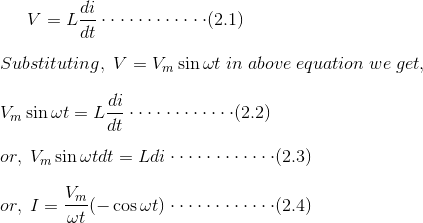Thus from above single phase power equation it is clear that I lags V by π/2 or in other words V leads I by π/2 , when AC pass through inductor i.e. I and V are out of phase as shown in fig (e).Instantaneous power is given by,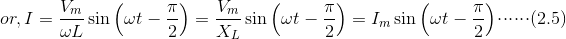Here, single phase power formula consists of only fluctuating term and the value of power for full cycle is zero.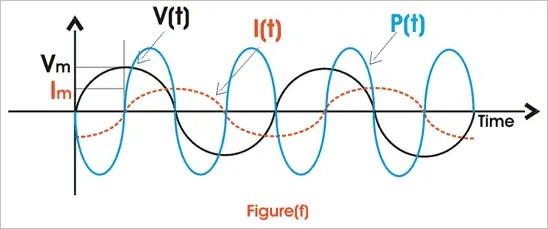### Equation for Purely Capacitive Circuit

When AC passes through capacitor, it charges first to its maximum value and then it discharges. The voltage across capacitor is given as,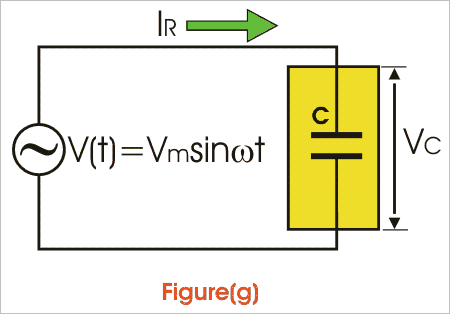Thus it is clear from above single phase power calculation of I(t) and V(t) that in case of capacitor current leads voltage by angle of π/2.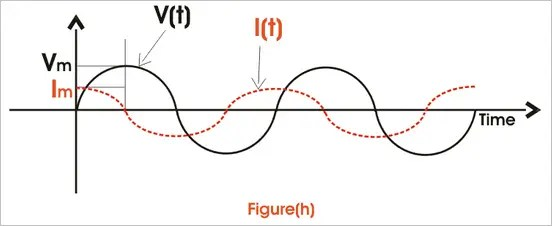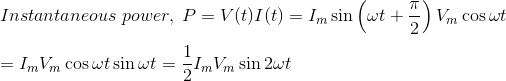Power through capacitor consists of only fluctuating term and the value of power for full cycle is zero.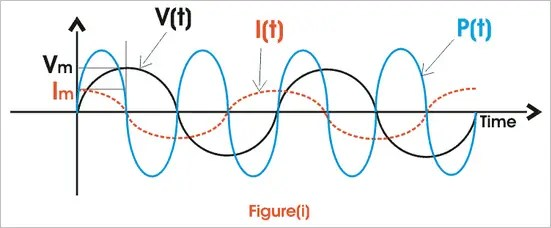### Single Phase Power Equation for RL Circuit

A pure ohmic resistor and inductor are connected in series below as shown in fig (g) across a voltage source V. Then drop across R will be VR = IR and across L will be VL = IXL.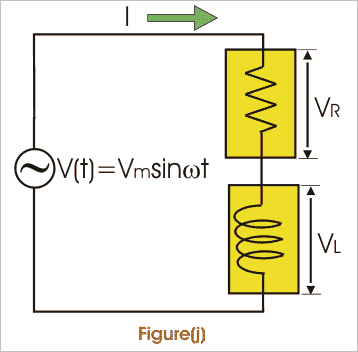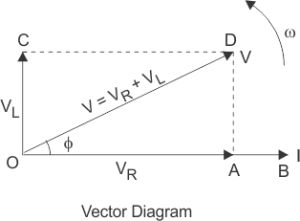These voltage drops are shown in form of a voltage triangle as shown in fig (i). Vector OA represents drop across R = IR, vector AD represents drop across L = IXL and vector OD represents the resultant of VR and VL.is the impedance of RL circuit.
From vector diagram it is clear that V leads I and phase angle φ is given by,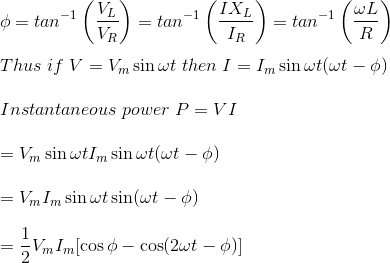Thus power consist of two terms, one constant term 0.5 VmImcosφ and other a fluctuating term 0.5 VmImcos(ωt – φ) that’s value is zero for the whole cycle.
Thus its the only constant part that contributes to actual power consumption.
Thus power, p = VI cos Φ = ( rms voltage × rms current × cosφ) watts
Where cosφ is called power factor and given by,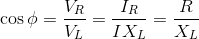I can be resolved in two rectangular components Icosφ along V and Isinφ perpendicular to V. Only Icosφ contributes to real power. Thus, only VIcosφ is called wattfull component or active component and VIsinφ is called wattless component or reactive component.

### Single Phase Power Equation for RC Circuit

We know that current in pure capacitance, leads voltage and in pure ohmic resistance it is in phase. Thus, net current leads voltage by angle of φ in RC circuit. If V = Vmsinωt and I will be Imsin(ωt + φ).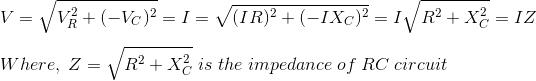Power is same as in the case of R-L circuit. Unlike R-L circuit electrical power factor is leading in R-C circuit.Scroll to Top
Scroll to Top